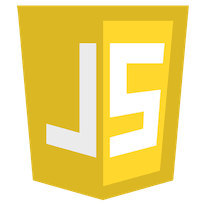I am currently looking for a software/web development job preferably as a Rails-based developer.### How To Shuffle An Array In Javascript

Posted by Weston Ganger

Creating a shuffle function for arrays can be complicated.

Here’s a 4 liner function for you that makes it really easy.

``````
function shuffle(o){
for(var j, x, i = o.length; i; j = Math.floor(Math.random() * i), x = o[--i], o[i] = o[j], o[j] = x);
return o;
}

shuffle(myArray);```
```